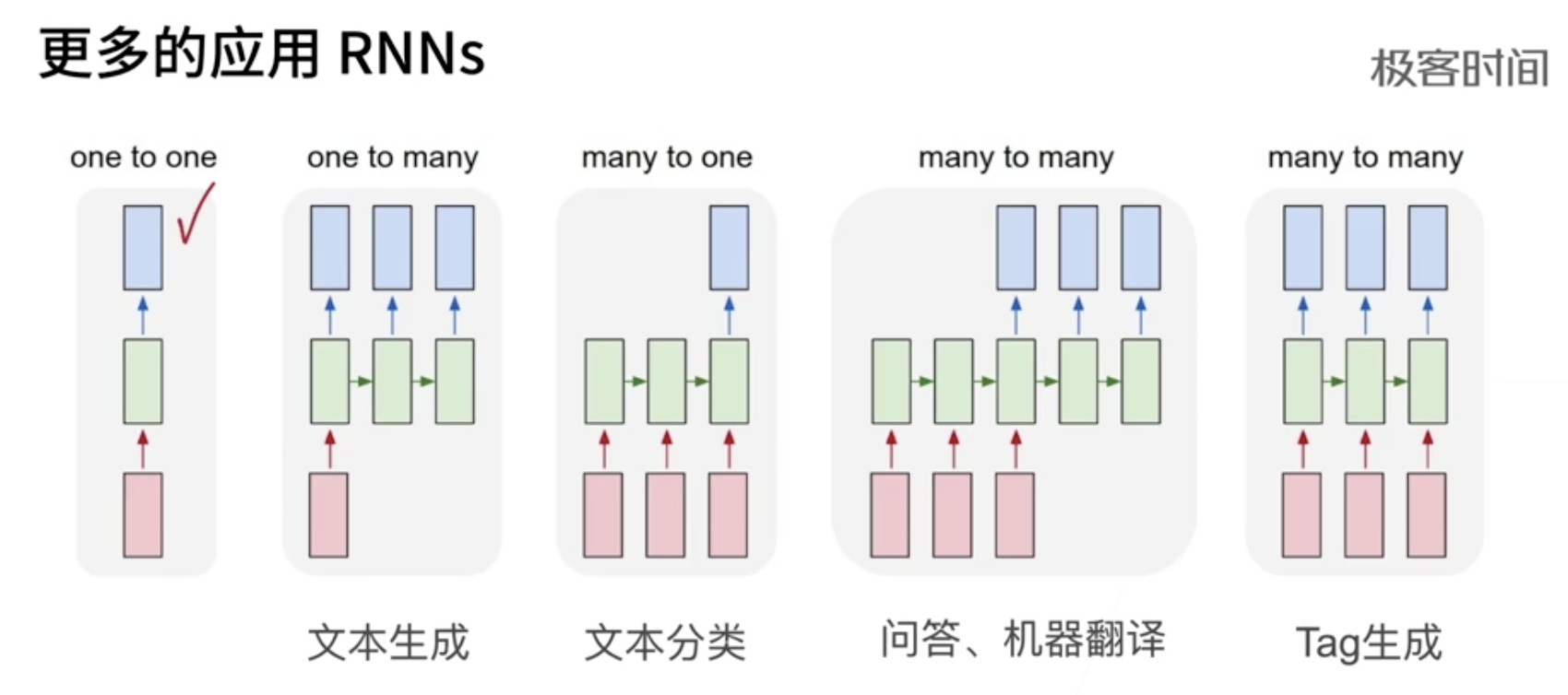2021年10月31日

## 简介（未完成）

1. 循环神经网络，引入状态变量来存储过去的信息，并用其与当期的输入共同决定当前的输出。 多层感知机 + 隐藏状态 = 循环神经网络
2. 应用到语言模型中时 ，循环神经网络根据当前词预测下一时刻词
3. 通常使用困惑度来衡量语言模型的好坏RNN 输入和输出 根据目的而不同

1. 比如 根据一个字预测下一个字，输入就是一个字的特征向量（后续就是这个字的某个数字编号）
2. 给一个词 标记是名词还是动词
3. 语音处理。输入一个每帧的声音信号 的特征向量

## 为什么要发明循环神经网络

1. 第一句话：I like eating apple！（我喜欢吃苹果！）
2. 第二句话：The Apple is a great company！（苹果真是一家很棒的公司！）## 字符集的循环神经网络 从零实现

``````def rnn(inputs, state, params):
# inputs和outputs皆为num_steps个形状为(batch_size, vocab_size)的矩阵
W_xh, W_hh, b_h, W_hq, b_q = params
H, = state
outputs = []
for X in inputs:
H = torch.tanh(torch.matmul(X, W_xh) + torch.matmul(H, W_hh) + b_h)  # H 是本次隐层的状态
Y = torch.matmul(H, W_hq) + b_q
outputs.append(Y)
return outputs, (H,)    # H 随本次 output 一起返回
def train_and_predict_rnn(rnn, get_params, init_rnn_state, num_hiddens,
vocab_size, device, corpus_indices, idx_to_char,
char_to_idx, is_random_iter, num_epochs, num_steps,
lr, clipping_theta, batch_size, pred_period,
pred_len, prefixes):
params = get_params()
loss = nn.CrossEntropyLoss()
for epoch in range(num_epochs):
l_sum, n, start = 0.0, 0, time.time()
data_iter = data_iter_fn(corpus_indices, batch_size, num_steps, device)
state = init_rnn_state(batch_size, num_hiddens, device)
for X, Y in data_iter:
inputs = to_onehot(X, vocab_size)
(outputs, state) = rnn(inputs, state, params)   # 核心就是这句
outputs = torch.cat(outputs, dim=0)
y = torch.transpose(Y, 0, 1).contiguous().view(-1)
l = loss(outputs, y.long())
# 梯度清0